## Subtraction Operator

Subtraction uses the `-` symbol, and is also known as minus.

How to subtract cells:

1. Select a cell and type (`=`)
2. Select the minuend
3. Type (`-`)
4. Select the subtrahend
5. Hit enter

Note: The minuend is the number to which the subtrahend subtracts from.

You can add more cells to the formula by typing (`-`) between the cells.

Let's have a look at some examples.

## Subtracting Two Manual Entries

Step by step:

1. Type `A1(=)`
2. Type `100-50`
3. Hit enter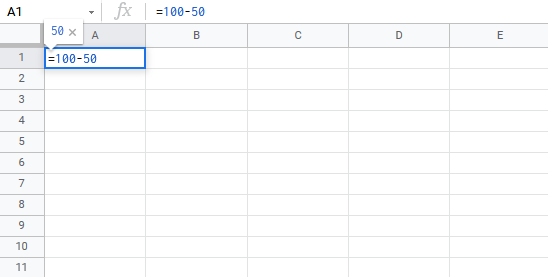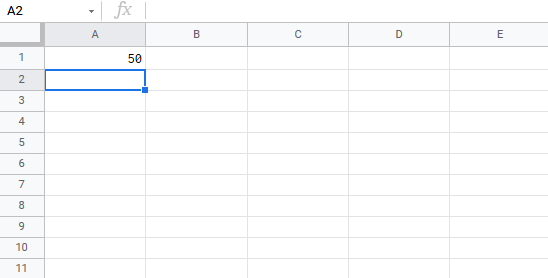Tip: You can add more values into the formula by typing (`-`) between the cells.

## Subtracting Using Two Cells

Let's add some numbers to work with. Type the following values: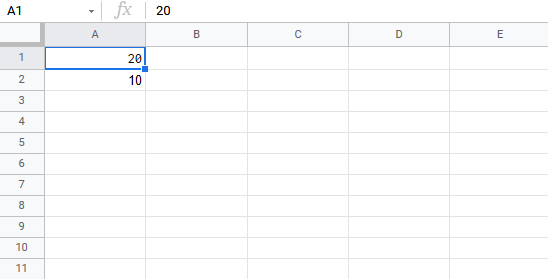Subtracting using two cells, step by step:

1. Type `A3(=)`
2. Select `A1`
3. Type (`-`)
4. Select `A2`
5. Hit enter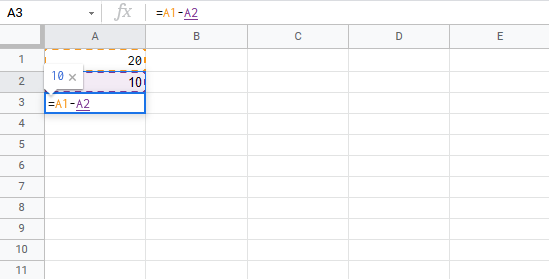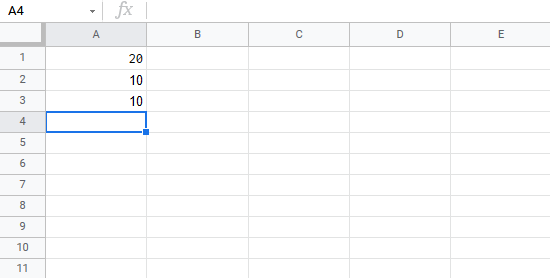## Subtracting Using Many Cells

Let's subtract using many cells. First, copy the following values: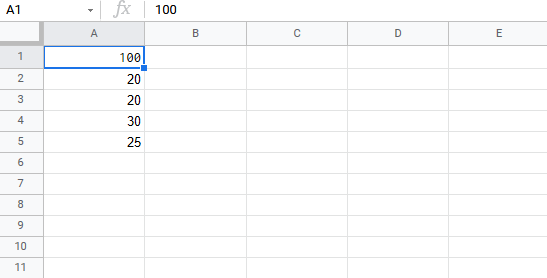Copy Values

Step by step:

1. Type `B1(=)`
2. Select `A1`
3. Type (`-`)
4. Select `A2`
5. Type (`-`)
6. Select `A3`
7. Type (`-`)
8. Select `A4`
9. Type (`-`)
10. Select `A5`
11. Hit enter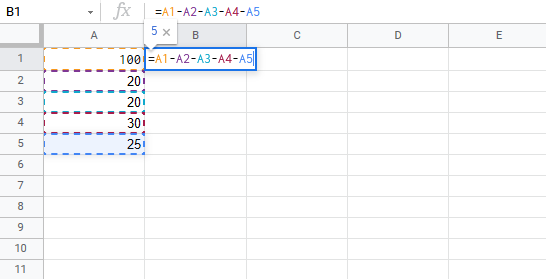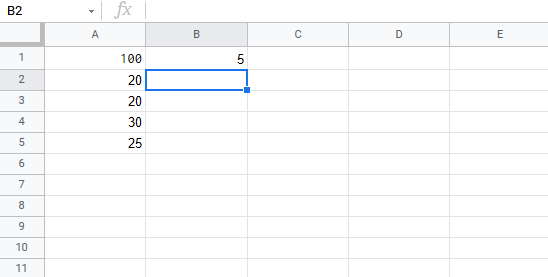## Subtracting Using Absolute Reference

You can lock a cell and subtract it from other cells.

How to do it, step by step:

1. Select a cell and type (`=`)
2. Select the minuend
3. Type (`-`)
4. Select the subtrahend and add two dollar signs (`\$`) before the column and row or press `F4`
5. Hit enter
6. Fill the range

Note: The minuend is the number to which the subtrahend subtracts from.

Let's have a look at an example where we subtract `B1(5)` from the range `A1:A10` using absolute reference and the fill function.

Copy the values to follow along: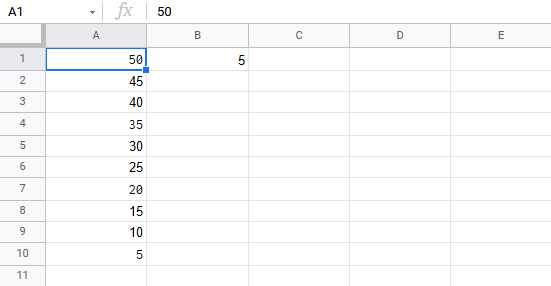Copy Values

Step by step:

1. Type `C1(=)`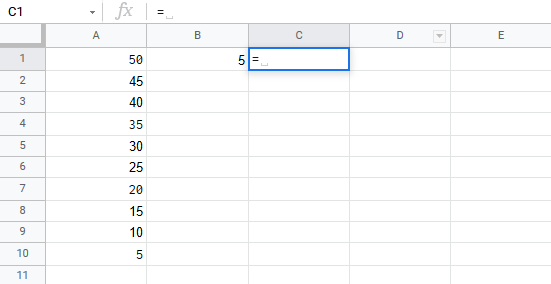1. Select `A1`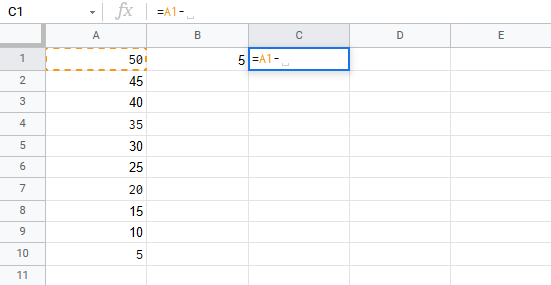1. Type (`-`)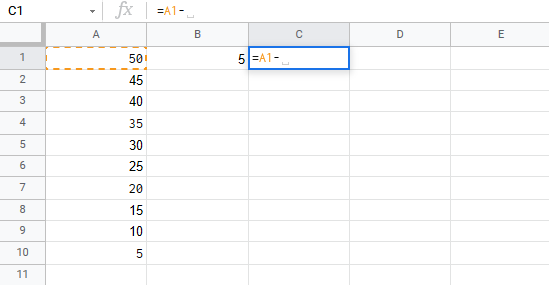1. Select `B1` and type dollar sign before column and row `\$B\$1`1. Hit Enter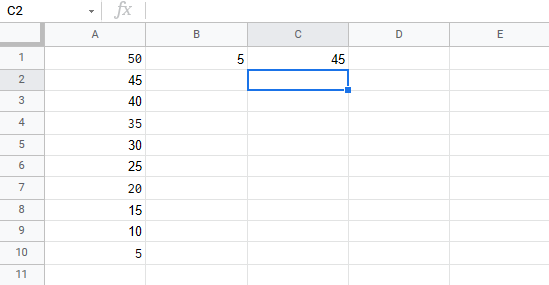1. Fill `C1:C10`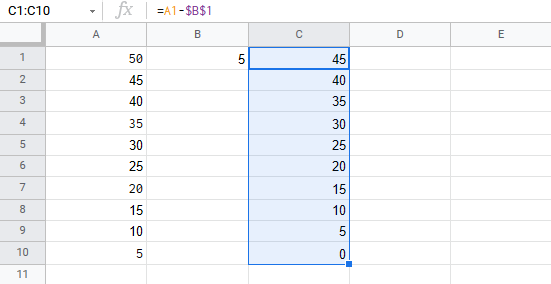You got it! You have successfully used absolute reference to subtract `B1(5)` from the minuend range `A1:A10`.

W3Schools is optimized for learning and training. Examples might be simplified to improve reading and learning. Tutorials, references, and examples are constantly reviewed to avoid errors, but we cannot warrant full correctness of all content. While using W3Schools, you agree to have read and accepted our terms of use, cookie and privacy policy.